# Distance problem 2

A=(x,2x)
B=(2x,1)
Distance AB=√2, find value of x

Correct result:

x1 =  1
x2 =  -0.2

#### Solution:

Our quadratic equation calculator calculates it.

$x_{2}=(-0.2)=- \dfrac{ 1 }{ 5 }=-0.2$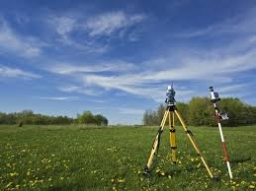We would be pleased if you find an error in the word problem, spelling mistakes, or inaccuracies and send it to us. Thank you!Tips to related online calculators
For Basic calculations in analytic geometry is a helpful line slope calculator. From coordinates of two points in the plane it calculate slope, normal and parametric line equation(s), slope, directional angle, direction vector, the length of segment, intersections the coordinate axes etc.
Looking for help with calculating roots of a quadratic equation?
Do you have a linear equation or system of equations and looking for its solution? Or do you have quadratic equation?
Do you want to convert length units?
Pythagorean theorem is the base for the right triangle calculator.

#### You need to know the following knowledge to solve this word math problem:

We encourage you to watch this tutorial video on this math problem:

## Next similar math problems:

• Parametric equationPoint A [6; -2]. Point B = [-3; 1] Write the parametric equation of the line BA so that t belongs to the closed interval 0; 3
• Perpendicular projectionDetermine the distance of a point B[1, -3] from the perpendicular projection of a point A[3, -2] on a straight line 2 x + y + 1 = 0.
• Ascend vs. descentWhich function is growing? a) y = 2-x b) y = 20 c) y = (x + 2). (-5) d) y = x-2
• General line equationsIn all examples, write the GENERAL EQUATION OF a line that is given in some way. A) the line is given parametrically: x = - 4 + 2p, y = 2 - 3p B) the line is given by the slope form: y = 3x - 1 C) the line is given by two points: A [3; -3], B [-5; 2] D) t
• Coordinates of the intersection of the diagonals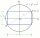In the rectangular coordinate system, a rectangle ABCD is drawn. The vertices of the rectangle are determined by these coordinates A = (2.2) B = (8.2) C = (8.6) D = (2.6) Find the coordinates of the intersection of the diagonals of the ABCD rectangle
• There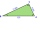There is a triangle ABC: A (-2,3), B (4, -1), C (2,5). Determine the general equations of the lines on which they lie: a) AB side, b) height to side c, c) Axis of the AB side, d) median ta to side a
• Isosceles triangleIn an isosceles triangle ABC with base AB; A [3,4]; B [1,6] and the vertex C lies on the line 5x - 6y - 16 = 0. Calculate the coordinates of vertex C.
• Find the 13Find the equation of the circle inscribed in the rhombus ABCD where A[1, -2], B[8, -3] and C[9, 4].
• Vector v4Find the vector v4 perpendicular to vectors v1 = (1, 1, 1, -1), v2 = (1, 1, -1, 1) and v3 = (0, 0, 1, 1)
• Calculate 6Calculate the distance of a point A[0, 2] from a line passing through points B[9, 5] and C[1, -1].
• Coordinates hexagon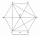The regular hexagon ABCDEF is given. Point A has coordinates [1; 3] and point D has coordinates [4; 7]. Calculate the sum of the coordinates of the center of its described circle.
• Chord BC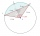A circle k has the center at the point S = [0; 0]. Point A = [40; 30] lies on the circle k. How long is the chord BC if the center P of this chord has the coordinates: [- 14; 0]?
• Vector perpendicularFind the vector a = (2, y, z) so that a⊥ b and a ⊥ c where b = (-1, 4, 2) and c = (3, -3, -1)
• Vector equationLet’s v = (1, 2, 1), u = (0, -1, 3) and w = (1, 0, 7) . Solve the vector equation c1 v + c2 u + c3 w = 0 for variables c1 c2, c3 and decide weather v, u and w are linear dependent or independent
• Angle of the body diagonalsUsing vector dot product calculate the angle of the body diagonals of the cube.
• Coordinates of a centroindLet’s A = [3, 2, 0], B = [1, -2, 4] and C = [1, 1, 1] be 3 points in space. Calculate the coordinates of the centroid of △ABC (the intersection of the medians).
• SupposeSuppose you know that the length of a line segment is 15, x2=6, y2=14 and x1= -3. Find the possible value of y1. Is there more than one possible answer? Why or why not?